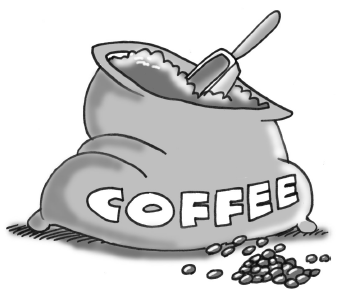Home > INT1 > Chapter 11 > Lesson 11.2.1 > Problem11-48

11-48.

How much coffee costing $6$ per pound should be mixed with $3$ pounds of coffee costing $4$ per pound to create a mixture costing $4.75$ per pound?Three pounds of $4/\text{lb}$ coffee is worth $12$. Let $x=\text{ the number of pounds of }\6/\text{lb coffee}$ .
Then $6x =\text{ the cost of that much coffee}$ . Put the total cost of the coffee (DOLLARS) over (PER) the total number of pounds (POUND) equal to $4.75$ per pound.
Then solve for $x$.

$\frac{\left(12+6\textit{x}\right)}{3+\textit{x}}=4.75$